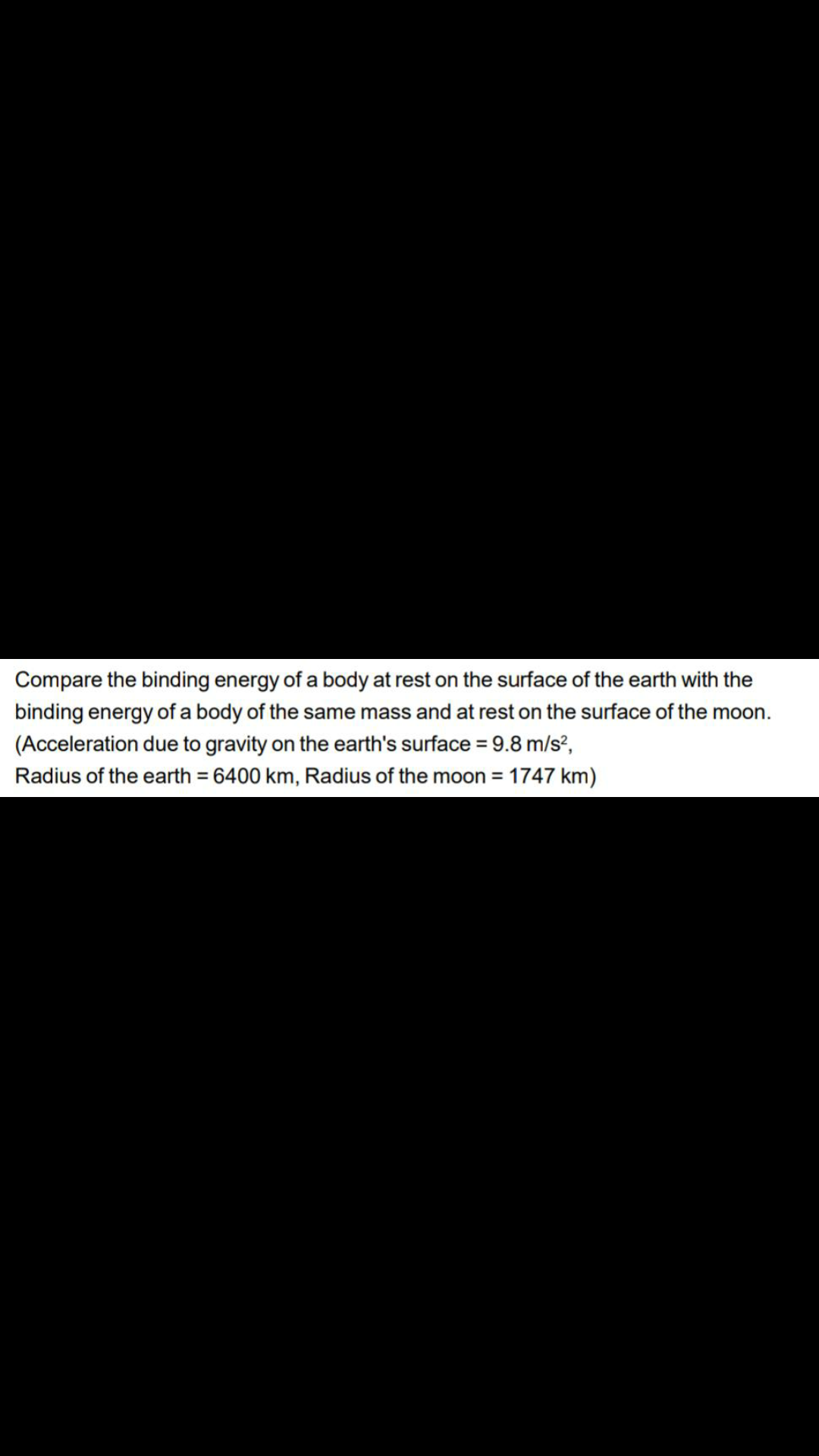# Compare the binding energy of a body at rest on the surface of the earth with the binding energy of a body of same mass at rest on the surface of the moon. (g = 9.8 m/s^2 , Radius of the earth = 6400 km, Radius of the moon= 1747 km)3 years ago

Binding energy on surface of earth is
Ee= GMem/Re = mgeRe

Similarly on moon it is
Em= GMmm/Rm = mgmRm

Comparing both
Ee/Em = geRe/gmRm
= 9.8 × 6400 / 1.625 × 1747
=22

It is 22 time more on earth than on moon